Courses

# VITEEE Chemistry Test - 6

## 40 Questions MCQ Test VITEEE: Subject Wise and Full Length MOCK Tests | VITEEE Chemistry Test - 6

Description
This mock test of VITEEE Chemistry Test - 6 for JEE helps you for every JEE entrance exam. This contains 40 Multiple Choice Questions for JEE VITEEE Chemistry Test - 6 (mcq) to study with solutions a complete question bank. The solved questions answers in this VITEEE Chemistry Test - 6 quiz give you a good mix of easy questions and tough questions. JEE students definitely take this VITEEE Chemistry Test - 6 exercise for a better result in the exam. You can find other VITEEE Chemistry Test - 6 extra questions, long questions & short questions for JEE on EduRev as well by searching above.
QUESTION: 1

Solution:
QUESTION: 2

Solution:
QUESTION: 3

### Schiff's reagent gives pink colour with

Solution:
QUESTION: 4

An organic compound 'A' has the molecular formula C₃H₆O. It undergoes iodoform test. When saturated with HCl it gives 'B' of molecular formula C₉H₁₄O. 'A' and 'B' respectively are

Solution:
QUESTION: 5

Propene when heated with chlorine at about 500oC forms

Solution:
QUESTION: 6

The typical reaction of olefinic bond is

Solution:
QUESTION: 7

Charring of sugar is due to

Solution:
QUESTION: 8

The helical structure of protein is stabilized by

Solution:
QUESTION: 9

Irrespective of the source, pure sample of water always yields 88.89% mass of oxygen and 11.11% mass of hydrogen. This is explained by the law of

Solution:
QUESTION: 10

How many monochlorobutanes will be obtained on chlorination of n-butane?

Solution: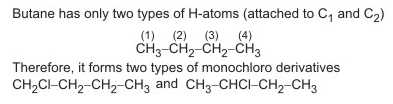QUESTION: 11

Lipids are

Solution:
QUESTION: 12

Which one of the following esters is obtained by the esterification of propan-2-ol with ethanoic acid?

Solution:
QUESTION: 13

A chemical reaction is catalyzed by a catalyst X. Hence X

Solution:
QUESTION: 14

A is a higher phenol and B is an aromatic carboxylic acid. Separation of a mixture of A and B can be carried out easily by having a solution

Solution:
QUESTION: 15

The final product 'C' in the above reaction is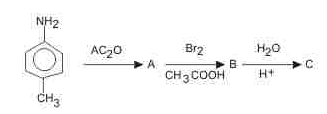Solution:
QUESTION: 16

A glass bulb is filled with NO₂ gas and immersed in an ice bath at 0oC which becomes colourless after some time . This colourless gas will be

Solution:
QUESTION: 17

An adiabatic expansion of an ideal gas always has

Solution:
QUESTION: 18

The Δ H value for the reaction H2 + Cl2 → 2HCl is − 44.12 K.cal. If E1 is the activation energy of the reactants and E2 is the activation energy of the products, then for the above reaction

Solution:
QUESTION: 19

Calculate the heat of formation Δ H of CO (in kcal) from the following data :
C(graphite) + O2 (g)  → CO2 (g)    ; Δ H = − 94 K . c a l
CO(g) + 1/2O2 (g)    → CO2 (g) ; Δ H = − 68 K . c a l

Solution:
QUESTION: 20
The Indian soils are generally poor in
Solution:
QUESTION: 21

Which is a pair of geometrical isomers ?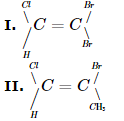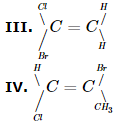r

Solution:
QUESTION: 22

The pair in which both species have the same magnetic moment (spin only value) is :

Solution:
QUESTION: 23

The IUPAC name for the complex [Co(NO₂)(NH₃)₅Cl₂] is

Solution:
QUESTION: 24

Hydrolysis of acetonitrile in acid medium gives

Solution:
QUESTION: 25

96500 C electricity is passed through CuSO₄. The amount of copper precipitated is

Solution:
QUESTION: 26

When benzenediazonium chloride in hydrochloric acid reacts with cuprous chloride, then chlorobenzene is formed. The reaction is called

Solution:
QUESTION: 27

The element, which forms oxides in all oxidation states + 1 to + 5, is

Solution:
QUESTION: 28

The e.m.f. of a galvanic cell with electrode potentials of Al equal to -1.66 V and that of Mg equal to - 0.54 V is

Solution:

E.M.F. can be positive only if oxidation takes place at Mg-electrode. But here the reduction reaction takes place.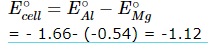QUESTION: 29

Which of the following metal is leached by cyanide process?

Solution:
QUESTION: 30

Acetic anhydride reacts with diethyl ether in the presence of anhydrous AlCl₃ to give

Solution:
QUESTION: 31

At which one of the following temperature-pressure conditions, the deviation of a gas from ideal behaviour is expected to be minimum ?

Solution:
QUESTION: 32

Which among CH₄, SiH₄, GeH₄ and SnH₄ is most volatile?

Solution:
QUESTION: 33

The oxidation state of nitrogen is highest in

Solution:
QUESTION: 34

Which of the following species is isoelectronic with CO ?

Solution:
QUESTION: 35

The process in which oxidation number increases is known as

Solution:
QUESTION: 36

The ratio of cationic radius to anionic radius in an ionic crystal is greater than 0.732. Its co-ordination number is

Solution:
QUESTION: 37

Maximum enolisation takes place of:

Solution:
QUESTION: 38

Adsorption is multiplayer in the case of

Solution:
QUESTION: 39

Which of the following metals exhibits more than one oxidation states?

Solution:

Out of the given alternatives Fe is only transition element.
Therefore it shows more than one oxidation state (+ 2 and + 3)
This is due to the fact that there is negligible energy difference
between outemost and penultimate shell

QUESTION: 40

An important ore of copper is

Solution: